# Quotient ring

(diff) ← Older revision | Latest revision (diff) | Newer revision → (diff)

of a ringby an idealThe quotient group of the additive group ofby the subgroup, with multiplication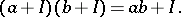The quotient turns out to be a ring and is denoted by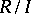. The mapping, where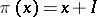, is a surjective ring homomorphism, called the natural homomorphism (see Algebraic system).

The most important example of a quotient ring is the ring of residues modulo— the quotient ring of the ring of integersby the ideal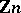. The elements of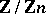can be assumed to be the numbers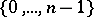, where the sum and the product are defined as the remainders on diving the usual sum and product by. One can establish a one-to-one order-preserving correspondence between the ideals ofand the ideals ofcontaining. In particular,is simple (cf. Simple ring) if and only ifis a maximal ideal.

Another most important example is the quotient ring, whereis the ring of polynomials overin one variableandis an irreducible polynomial. This quotient ring describes all field extensions ofby roots of the equation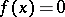(cf. also Extension of a field).